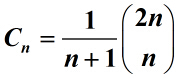# Catalan数在一类特殊的数学结构计数的应用Applications of Catalan Number in a Special Mathematical Structure Counting

DOI: 10.12677/AAM.2016.53047, PDF, HTML, XML, 下载: 1,328  浏览: 3,010  国家自然科学基金支持

Abstract: Catalan number is an important counting function in the combination of a counting theory. It’s general formula:. It was first used by the mathematician Antu in Qing dynasty. The paper uses the method of Catalan number and generating function to solve a class of problems counting the special mathematical structure and constructs the explicit expression of the solution of the mathematical structure. Finally, the paper gives another solution of the mathematical structure counting problem and also makes use of generalized Catalan number from another angle to solve the problem.

  罗见今. 明安图是卡特兰数的首创者[J]. 内蒙古大学学报, 1998, 19(2): 239-245.  刘芹英. 经典Catalan数的组合背景[J]. 西北大学学报. 2003, 33(1): 121-124.  初文昌. 关于Catalan序列[J]. 大连铁道学院, 1985, 6(4): 7-21.  徐海涛. 广义Catalan矩阵及其组合意义[J]. 甘肃科学学报, 2013, 27(3): 13-15.  张勇. Catalan数与二项式系数和式的同余式[J]. 数学进展, 2014, 43(6): 857-862.  王丽娟, 杨胜良. Riordan矩阵在广义Motzkin路计数中的应用[J]. 纯粹数学与应用数学, 2016, 32(2): 160-168.  赵浩仿. 指数Riordan群的一些性质及应用[J]. 滨州学院学报, 2013, 29(3): 73-77.  林翠琴. 组合学与图论[M]. 北京: 清华大学出版社, 2009.  Fellew, W. (1957) An Introduction to Probability Theory and Its Applications. 2nd Edition, Wiley, New York.